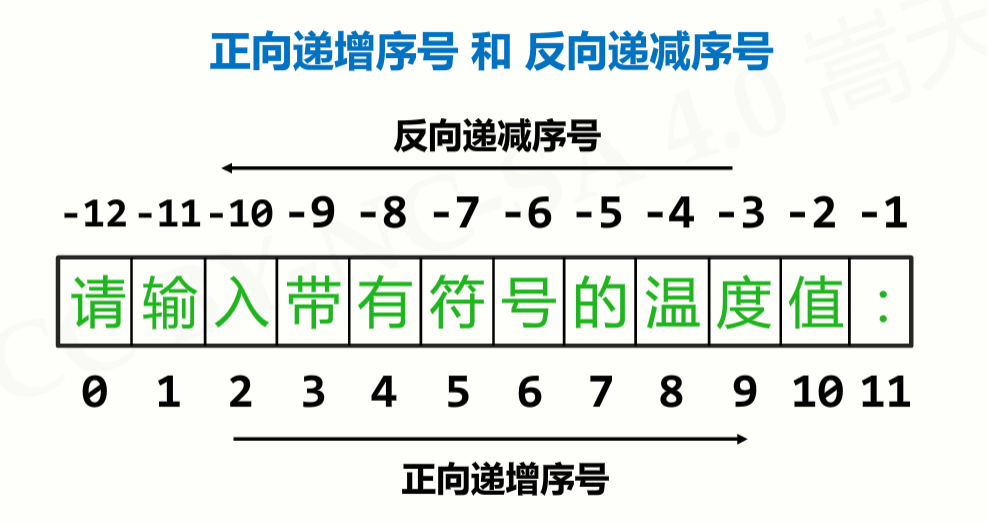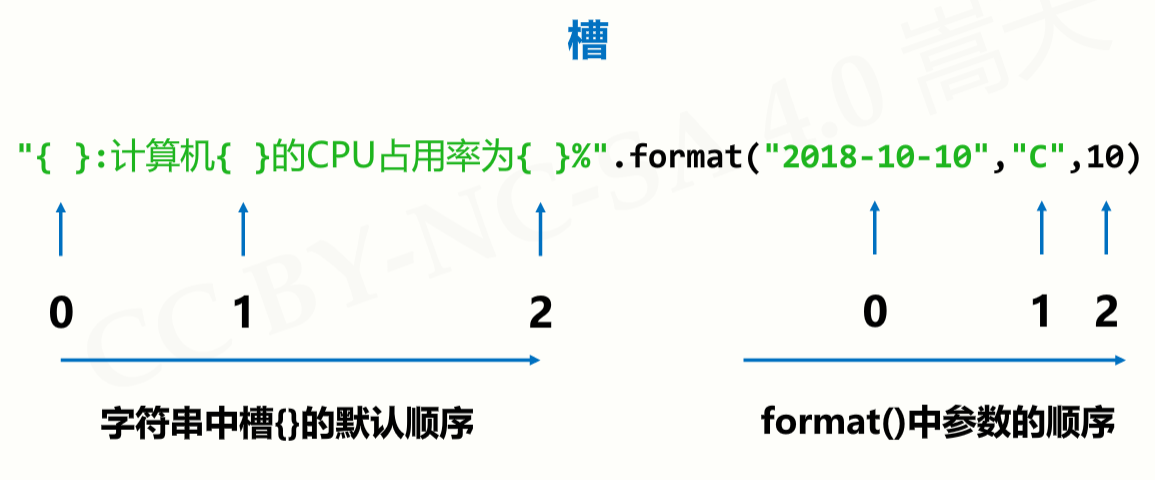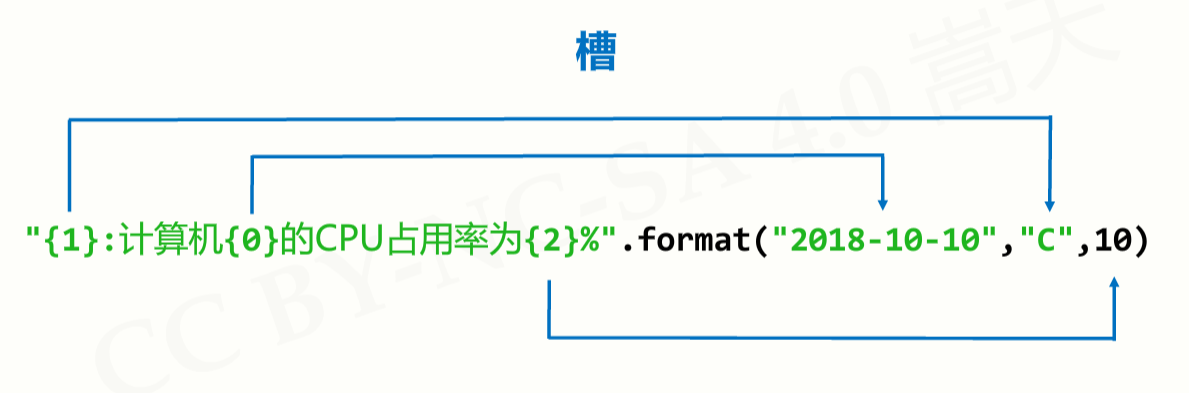# 输入

`input("提示性信息")`

`input("请输入数字")`

## 评估函数

`eval("1+2")` → 3
`eval('"1+2"')` → ‘1+2’
`eval('print("hello")')` → hello

# 输出

`print(...)`

`print(...., end = "")`

# 数字类型

## 整数类型

• 可正可负，没有取值范围限制
• `pow(x, y)` 函数：计算\(x^y\)，想算多大算多大

• 十进制：1010，99，-217
• 二进制：以 0b 或 0B 开头：0b010，-0B101
• 八进制：以 0o 或 0O 开头：0o123，-0O456
• 十六进制：以 0x 或 0X 开头：0x9a，-0X89

## 浮点数类型

• 带有小数点及小数的数字
• 浮点数取值范围和小数精度都存在限制，但常规计算可忽略
• 取值范围数量级约为\(-10^{307}\)\(10^{308}\)，精度数量级\(10^{-16}\)
• 浮点数间运算存在不确定尾数，不是 bug

### 不确定尾数

0.1+0.2 → 0.30000000000000004

• 二进制表示：0.00011001100110011001100110011001100…等 53位二进制表示小数部分，约 \(10^{-16}\)
• 十进制表示：0.10000000000000000555111512312578270…

注意：二进制表示小数，可以无限接近，但不完全相同。
例如，0.1+0.2 结果无限趋近 0.3，但是可能存在尾数。

### 四舍五入

• 0.1+0.2 == 0.3 → False
• round(0.1+0.2, 1) == 0.3 → True

• `round(x, d)`：对 x 四舍五入，d 是小数截取位数。
• 浮点数间运算与比较用 round() 函数辅助
• 不确定尾数一般发生在 \(10^{-16}\) 左右，round() 十分有效

### 科学计数法

• 使用字母 e 或 E 作为幂的符号，以 10 为基数，格式如下：
`<a>e<b>` 表示 \(a*10^b\)

例如：4.3e-3 值为 0.0043
9.6E5 值为 960000.0

## 复数类型

z.real 获得实部，z.imag 获得虚部

## 数值运算操作符

x // y 整数除 x 与 y之整数商 10//3 结果是 3
x % y 余数，模运算 10%3 结果是 1
x ** y 幂运算，x的y次幂，\(x^y\) 也可以进行开方
+x x 的本身
-x x 的负值

## 数值运算函数

abs(x) 绝对值，x 的绝对值 abs(-10.01) 结果为 10.01
divmod(x, y) 商余，(x//y, x%y)，同时输出商和余数 divmod(10, 3) 结果为 (3,1)
pow(x, y[,z]) 幂余，(x**y%z)，[]表示参数z可省略 pow(3, pow(3,99),10000) 结果为 4587
round(x[,d]) 四舍五入，d 是保留小数位数，默认值为 0 round(-10.123,2) 结果为 -10.12
max(x1,x2,…,xn) 最大值，返回 x1,x2,…,xn 中的最大值，n 不限 max(1,9,5,4,3) 结果为 9
min(x1,x2,…,xn) 最小值，返回 x1,x2,…,xn 中的最小值，n 不限 min(1,9,5,4,3) 结果为 1
int(x) 将 x 变成整数，舍弃小数部分 int(123.45)结果为123；
int(“123”)结果为123
float(x) 将 x 变成浮点数，增加小数部分 float(12)结果为12.0；
float(“1.23”)结果为1.23
complex(x) 将 x 变成复数，增加虚数部分 complex(4)结果为4+0j

# 字符串类型

## 字符串类型的表示

• 字符串由一对单引号或一对双引号表示

例如：“请输入带有符号的温度值：” 或者 ‘C’

• 字符串是字符的有序序列，可以对其中的字符进行索引

例如：“请”是”请输入带有符号的温度值：”的第 0 个字符

• 由一对单引号或双引号表示，仅表示单行字符串

例如：“请输入带有符号的温度值：” 或者 ‘C’

• 由一对三单引号或三双引号表示，课表示多行字符串

例如：
”’
python
语言
”’

• 如果希望在字符串中包含双引号或单引号呢？
‘这里有个双引号(“)’ 或者 “这里有个单引号(‘)”
• 如果希望在字符串中既包括单引号又包括双引号呢？
”’这里既有单引号(‘)又有双引号(“)”’

### 字符串的序号### 字符串的使用

• 索引：返回字符串中单个字符。<字符串>[M]

例如：“请输入带有符号的温度值：” 或者 TempStr[-1]

• 切片：返回字符串中一段字符子串。<字符串>[M:N]

例如：“请输入带有符号的温度值：”[1:3] 或者 TempStr[0:-1]

#### 字符串切片高级用法

• <字符串>[M:N]，M 缺失表示至开头N 缺失表示至结尾

例如：“零一二三四五六七八九十”[:3] 结果是 “零一二”

• <字符串>[M:N:K]，根据步长 K 对字符串切片

例如：“零一二三四五六七八九十”[1:8:2] 结果是 “一三五七”
“零一二三四五六七八九十”[::-1] 结果是 “十九八七六五四三二一零”

## 字符串操作符

x + y 连接两个字符串 x 和 y
x*n 或 n*x 复制 n 次字符串 x
x in s 如果 x 是 s 的子串，返回 True，否则返回 False

## 字符串处理函数

len(x) 长度，返回字符串 x 的长度 len(“一二三456”)结果为6
str(x) 任意类型 x 所对应的字符串形式 str(1.23)结果为”1.23″
str([1,2])结果为”[1,2]”
oct(x) 整数 x 的八进制小写形式字符串 oct(425)结果为”0o651″
hex(x) 整数 x 的十六进制小写形式字符串 hex(425)结果为”0x1a9″
chr(u) x 为 Unicode 编码，返回其对应的单字符
ord(x) x 为字符，返回其对应的 Unicode编码

## 字符串处理方法

str.lower() 返回字符串的副本，全部字符小写 “AbCdEfGh”.lower()结果为”abcdefgh”
str.upper() 返回字符串的副本，全部字符大写
strsplit(sep=None) 返回一个列表，由 str 根据 sep 被分隔的部分组成 “A,B,C”.split(“,”)结果为[‘A’,’B’,’C’]
str.count(sub) 返回子串 sub 在 str 中出现的次数 “an apple a day”.count(“a”)结果为4
str.replace(old, new) 返回字符串 str 副本，所有 old 子串被替换为 new “python”.replace(“n”, “n123.io”)结果为”python123.io”
str.center(width[,fillchar]) 字符串 str 根据宽度 width 居中，fillchar 可选 “python”.center(20,”=”)结果为”=======python=======”
str.strip(chars) 从 str 中去掉在其左侧和右侧 chars中列出的字符 “= python=”.strip(” =np”)结果为”ytho”
str.join(iter) 在 iter 变量除最后元素外每个元素后增加一个 str “,”.join(“12345″)结果为”1,2,3,4,5”

## 字符串类型的格式化

• 字符串格式化使用.format()方法，用法如下：
<模板字符串>.format(<逗号分隔的参数>)### 槽内部对格式化的配置方式

{<参数序号>:<格式控制标记>}

: <填充> <对齐> <宽度> <,> <.精度> <类型>

`>` 右对齐
`^` 居中对齐

`b`,`c`,`d`,`o`,`x`,`X`

`e`,`E`,`f`,`%`

`"{0:=^20}".format("PYTHON")`
→ ‘=======PYTHON=======’
`"{0:*>20}".format("BIT")`
→ ‘*****************BIT’
`"{:10}".format("BIT")`
‘BIT ‘

`"{0:,.2f}".format(12345.6789)`
→ ‘12,345.68’
`"{0:b},{0:c},{0:d},{0:o},{0:x},{0:X}x".format(425)`
→ ‘110101001,Σ,425,651,1a9,1A9’
`"{0:e},{0:E},{0:f},{0:%}".format(3.14)`
‘3.140000e+00,3.140000E+00,3.140000,314.000000%’

# 异常处理

``````try:
# 执行1
<语句块1>
except [<异常类型>]:
# 如果出现异常执行2
<语句块2>
[else:]
# 否则，不发生异常执行3
<语句块3>
[finally:]
# 最后执行4，一定执行
<语句块4>``````

`raise [Exception [, args [, traceback]]]`

# 分支结构

## 二分支结构

`<表达式1> if <条件> else <表达式2>`

``````guess = eval(input())
print("猜{}了".format("对" if guess==99 else "错"))``````

## 多分支结构

``````if
elif
else``````

# 循环结构

## 遍历循环

``````for <循环变量> in <遍历结构> :
<语句块> ``````
• 从遍历结构中逐一提取元素，放在循环变量中
• 由保留字for和in组成，完整遍历所有元素后结束
• 每次循环，所获得元素放入循环变量，并执行一次语句块

### 计数循环（N次）

``````for i in range(N) :
<语句块> ``````
• 遍历由`range()`函数产生的数字序列，产生循环

``````for i in range(5):
print("Hello:",i)
``````

``````Hello: 0
Hello: 1
Hello: 2
Hello: 3
Hello: 4``````

### 计数循环（特定次）

``````for i in range(M,N,K) :
<语句块> ``````
• 遍历由`range()`函数产生的数字序列，产生循环

``````for i in range(1,6,2):
print("Hello:",i)``````

``````Hello: 1
Hello: 3
Hello: 5``````

### 字符串遍历循环

``````for c in s :
<语句块> ``````
• s是字符串，遍历字符串每个字符，产生循环

``````for c in "Python123":
print(c, end=",")``````

``P,y,t,h,o,n,1,2,3,``

### 列表遍历循环

``````for item in ls :
<语句块> ``````
• ls是一个列表，遍历其每个元素，产生循环

``````for item in [123, "PY", 456] :
print(item, end=",")``````

``123,PY,456,``

### 文件遍历循环

``````for line in fi :
<语句块> ``````
• fi是一个文件标识符，遍历其每行，产生循环

``````for line in fi :
print(line)``````

``````优美胜于丑陋

## 无限循环

``````while <条件> :
<语句块> ``````
• 反复执行语句块，直到条件不满足时结束

``````a = 3
while a > 0 :
a = a - 1
print(a)``````

``````2
1
0``````

## 扩展

``````for <变量> in <遍历结构> :
<语句块1>
else :
<语句块2>``````
``````while <条件> :
<语句块1>
else :
<语句块2>``````
• 当循环没有被break语句退出时，执行else语句块
• else语句块作为”正常”完成循环的奖励
• 这里else的用法与异常处理中else用法相似

``````for c in "PYTHON" :
if c == "T" :
continue
print(c, end="")
else:
print("正常退出")``````

``PYHON正常退出``

``````for c in "PYTHON" :
if c == "T" :
break
print(c, end="")
else:
print("正常退出")``````

``PY``

# 函数

• 函数定义时可以为某些参数指定默认值，构成可选参数
• 函数定义时可以设计可变数量参数，即 不确定参数总数量
• 函数调用时，参数可以按照位置名称方式传递，如 f(1, 2) → f(m=1, n=2)
• 函数可以返回 0 个或多个结果（元组类型）
``````def <函数名>(<非可选参数> [,<可选参数>, <可变参数>]) :
<函数体>
return <返回值>``````

``````def f(m, n=1)
return m+n

print(f(1))``````

``2``

``````def f(*b):
sum = 0
for item in b:
sum += item
return sum

print(f(1,2,3,4,5))``````

``15``

（事实上在函数中，和才是必要的，args 和 kwargs 可以用其他名称代替）
*args 是指不定数量的非键值对参数。
**kwargs 是指不定数量的键值对参数。

*args 作为作为元组匹配没有指定参数名的参数。而 **kwargs 作为字典，匹配指定了参数名的参数。
*args 必须位于 **kwargs 之前。

*args(*通常紧跟一个标识符，你会看到a或者args都是标识符)是python用于接收或者传递任意基于位置的参数的语法。当你接收到一个用这种语法描叙参数时（比如你在函数def语句中对函数签名使用了星号语法），python会将此标识符绑定到一个元祖，该元祖包含了所有基于位置的隐士的接收到的参数。当你用这种语法传递参数时，标识符可以被绑定到任何可迭代对象（事实上，它也可以是人和表达式，并不必须是一个标识符），只要这个表达式的结果是一个可迭代的对象就行。

**kwds(标识符可以是任意的，通常k或者kwds表示)是python用于接收或者传递任意基于位置的参数的语法。（python有时候会将命名参数称为关键字参数，他们其实并不是关键字–只是用他们来给关键字命名，比如pass,for或者yield，还有很多，不幸的是，这种让人疑惑的术语目前仍是这门语言极其文化根深蒂固的一个组成部分。）当你接收到用这种语法描叙的一个参数时（比如你在函数的def语句中对函数签名使用了双星号语法）python会将标识符绑定到一个字典，该字典包含了所有接收到的隐士的命名参数。当你用这种语法传递参数时，标识符只能被绑定到字典（我ID号I它也可以是表达式，不一定是一个标识符，只要这个表达式的结果是一个字典即可）。

## 局部变量和全局变量

• 基本数据类型，无论是否重名，局部变量与全局变量不同
• 可以通过 global 保留字在函数内部声明全局变量
• 组合数据类型，如果局部变量未真实创建，则是全局变量

解释：组合数据类型是用指针来指明位置的，所以若局部变量未真实创建组合数据类型，它使用的变量是指针，而指针指的是外部的全局变量，所以你去修改指针对应的内容就修改了全局变量。

## lambda 函数

lambda函数返回函数名作为结果

• lambda函数是一种匿名函数，即没有名字的函数
• 使用lambda保留字定义，函数名是返回结果
• lambda函数用于定义简单的、能够在一行内表示的函数
``````<函数名> = lambda <参数>: <表达式>

def <函数名>(<参数>) :
<函数体>
return <返回值>``````

``````f = lambda : "lambda函数"
print(f())``````

``lambda函数``

• lambda函数主要用作一些特定函数或方法的参数
• lambda函数有一些固定使用方式，建议逐步掌握
• 一般情况，建议使用def定义的普通函数